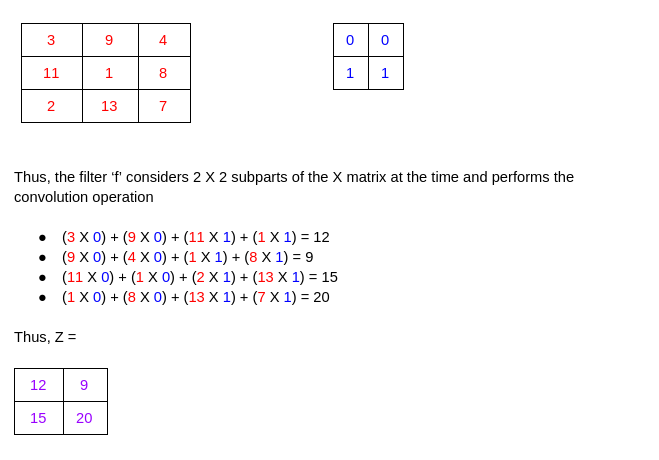# Why does a Convolutional Neural Network (CNN) work better with image data?

Q:
Why does a Convolutional Neural Network (CNN) work better with image data?

## ▼ Show 1 Answer

0 votes

The key to this question lies in the Convolution operation. Unlike humans, the machine sees the image as a matrix of pixel values. Instead of interpreting a shape like a petal or an ear, it just identifies curves and edges.

Thus, instead of looking at the entire image, it helps to just read the image in parts. Doing this for a 300 x 300 pixel image would mean dividing the matrix into smaller 3 x 3 matrices and dealing with them one by one. This is convolution.

Mathematically, we just perform a small operation on the matrix to help us detect features in the image – like boundaries, colors, etc.

Z = X * f

Here, we are convolving (* operation – not multiplication) the input matrix X with another small matrix f, called the kernel/filter to create a new matrix Z. This matrix is then passed on to the other layers.

If you have a board/screen in front of you, you can always illustrate this with a simple example:Learn More with Madanswer

0 votes
0 votes
0 votes
0 votes
+1 vote
0 votes
0 votes